Anzeige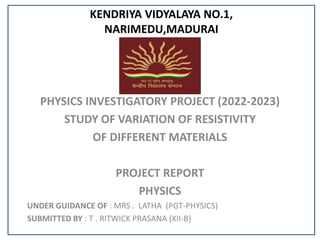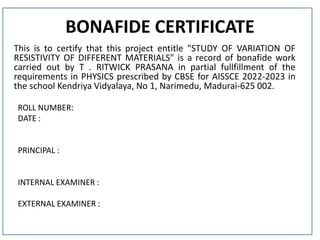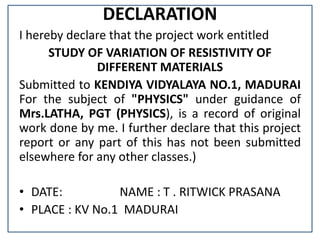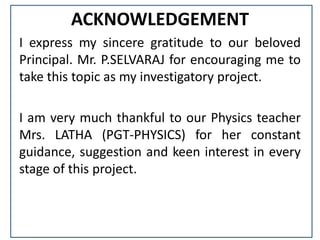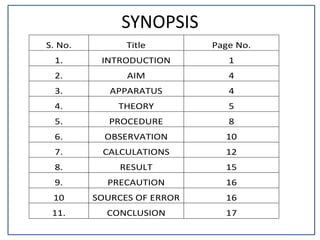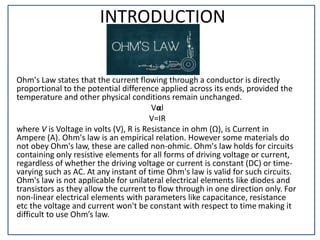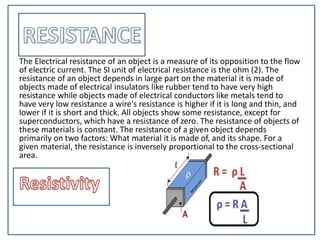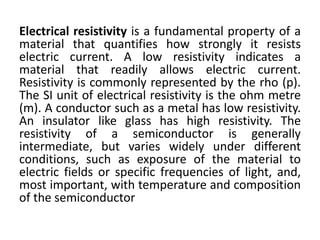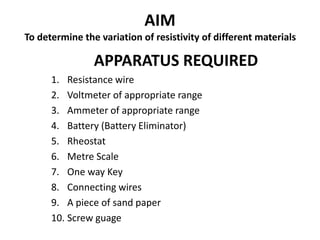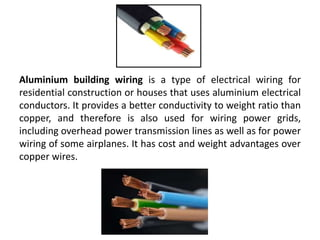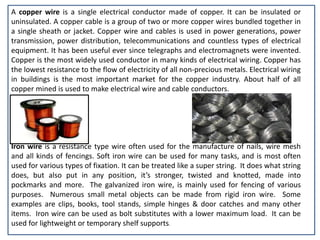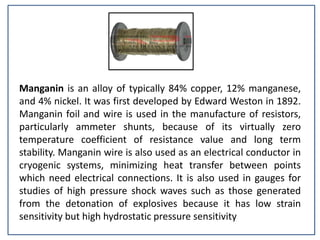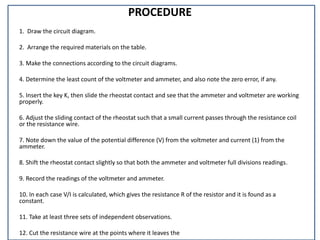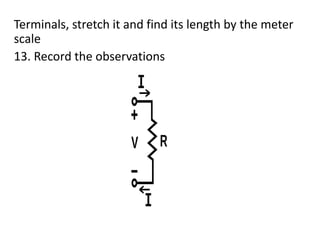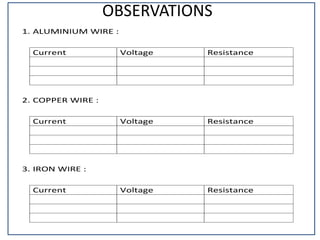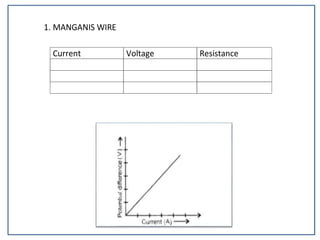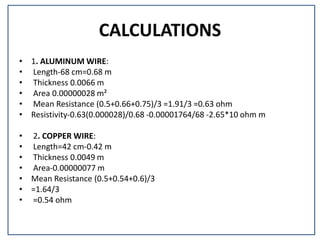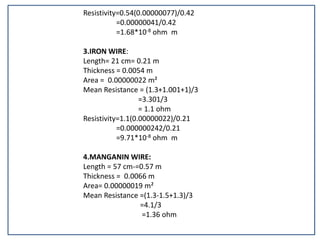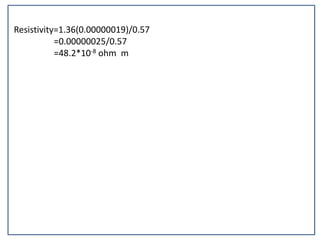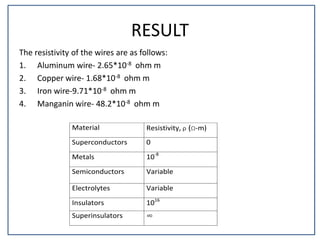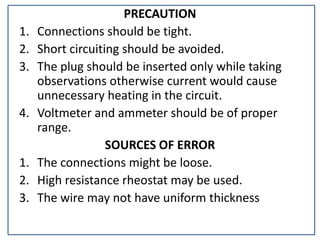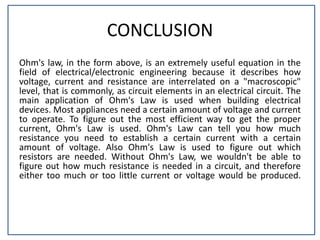1 von 23
Anzeige

### PHYSICS PROJECT RITWICK.pptx

1. KENDRIYA VIDYALAYA NO.1, NARIMEDU,MADURAI PHYSICS INVESTIGATORY PROJECT (2022-2023) STUDY OF VARIATION OF RESISTIVITY OF DIFFERENT MATERIALS PROJECT REPORT PHYSICS UNDER GUIDANCE OF : MRS . LATHA (PGT-PHYSICS) SUBMITTED BY : T . RITWICK PRASANA (XII-B)
2. BONAFIDE CERTIFICATE This is to certify that this project entitle "STUDY OF VARIATION OF RESISTIVITY OF DIFFERENT MATERIALS" is a record of bonafide work carried out by T . RITWICK PRASANA in partial fullfillment of the requirements in PHYSICS prescribed by CBSE for AISSCE 2022-2023 in the school Kendriya Vidyalaya, No 1, Narimedu, Madurai-625 002. ROLL NUMBER: DATE : PRINCIPAL : INTERNAL EXAMINER : EXTERNAL EXAMINER :
3. DECLARATION I hereby declare that the project work entitled STUDY OF VARIATION OF RESISTIVITY OF DIFFERENT MATERIALS Submitted to KENDIYA VIDYALAYA NO.1, MADURAI For the subject of "PHYSICS" under guidance of Mrs.LATHA, PGT (PHYSICS), is a record of original work done by me. I further declare that this project report or any part of this has not been submitted elsewhere for any other classes.) • DATE: NAME : T . RITWICK PRASANA • PLACE : KV No.1 MADURAI
4. ACKNOWLEDGEMENT I express my sincere gratitude to our beloved Principal. Mr. P.SELVARAJ for encouraging me to take this topic as my investigatory project. I am very much thankful to our Physics teacher Mrs. LATHA (PGT-PHYSICS) for her constant guidance, suggestion and keen interest in every stage of this project.
5. SYNOPSIS S. No. Title Page No. 1. INTRODUCTION 1 2. AIM 4 3. APPARATUS 4 4. THEORY 5 5. PROCEDURE 8 6. OBSERVATION 10 7. CALCULATIONS 12 8. RESULT 15 9. PRECAUTION 16 10 SOURCES OF ERROR 16 11. CONCLUSION 17
6. INTRODUCTION Ohm's Law states that the current flowing through a conductor is directly proportional to the potential difference applied across its ends, provided the temperature and other physical conditions remain unchanged. Vαl V=IR where V is Voltage in volts (V), R is Resistance in ohm (Ω), is Current in Ampere (A). Ohm's law is an empirical relation. However some materials do not obey Ohm's law, these are called non-ohmic. Ohm's law holds for circuits containing only resistive elements for all forms of driving voltage or current, regardless of whether the driving voltage or current is constant (DC) or time- varying such as AC. At any instant of time Ohm's law is valid for such circuits. Ohm's law is not applicable for unilateral electrical elements like diodes and transistors as they allow the current to flow through in one direction only. For non-linear electrical elements with parameters like capacitance, resistance etc the voltage and current won't be constant with respect to time making it difficult to use Ohm’s law.
7. The Electrical resistance of an object is a measure of its opposition to the flow of electric current. The SI unit of electrical resistance is the ohm (2). The resistance of an object depends in large part on the material it is made of objects made of electrical insulators like rubber tend to have very high resistance while objects made of electrical conductors like metals tend to have very low resistance a wire's resistance is higher if it is long and thin, and lower if it is short and thick. All objects show some resistance, except for superconductors, which have a resistance of zero. The resistance of objects of these materials is constant. The resistance of a given object depends primarily on two factors: What material it is made of, and its shape. For a given material, the resistance is inversely proportional to the cross-sectional area.
8. Electrical resistivity is a fundamental property of a material that quantifies how strongly it resists electric current. A low resistivity indicates a material that readily allows electric current. Resistivity is commonly represented by the rho (p). The SI unit of electrical resistivity is the ohm metre (m). A conductor such as a metal has low resistivity. An insulator like glass has high resistivity. The resistivity of a semiconductor is generally intermediate, but varies widely under different conditions, such as exposure of the material to electric fields or specific frequencies of light, and, most important, with temperature and composition of the semiconductor
9. AIM To determine the variation of resistivity of different materials APPARATUS REQUIRED 1. Resistance wire 2. Voltmeter of appropriate range 3. Ammeter of appropriate range 4. Battery (Battery Eliminator) 5. Rheostat 6. Metre Scale 7. One way Key 8. Connecting wires 9. A piece of sand paper 10. Screw guage
10. Aluminium building wiring is a type of electrical wiring for residential construction or houses that uses aluminium electrical conductors. It provides a better conductivity to weight ratio than copper, and therefore is also used for wiring power grids, including overhead power transmission lines as well as for power wiring of some airplanes. It has cost and weight advantages over copper wires.
11. A copper wire is a single electrical conductor made of copper. It can be insulated or uninsulated. A copper cable is a group of two or more copper wires bundled together in a single sheath or jacket. Copper wire and cables is used in power generations, power transmission, power distribution, telecommunications and countless types of electrical equipment. It has been useful ever since telegraphs and electromagnets were invented. Copper is the most widely used conductor in many kinds of electrical wiring. Copper has the lowest resistance to the flow of electricity of all non-precious metals. Electrical wiring in buildings is the most important market for the copper industry. About half of all copper mined is used to make electrical wire and cable conductors. Iron wire is a resistance type wire often used for the manufacture of nails, wire mesh and all kinds of fencings. Soft iron wire can be used for many tasks, and is most often used for various types of fixation. It can be treated like a super string. It does what string does, but also put in any position, it’s stronger, twisted and knotted, made into pockmarks and more. The galvanized iron wire, is mainly used for fencing of various purposes. Numerous small metal objects can be made from rigid iron wire. Some examples are clips, books, tool stands, simple hinges & door catches and many other items. Iron wire can be used as bolt substitutes with a lower maximum load. It can be used for lightweight or temporary shelf supports.
12. Manganin is an alloy of typically 84% copper, 12% manganese, and 4% nickel. It was first developed by Edward Weston in 1892. Manganin foil and wire is used in the manufacture of resistors, particularly ammeter shunts, because of its virtually zero temperature coefficient of resistance value and long term stability. Manganin wire is also used as an electrical conductor in cryogenic systems, minimizing heat transfer between points which need electrical connections. It is also used in gauges for studies of high pressure shock waves such as those generated from the detonation of explosives because it has low strain sensitivity but high hydrostatic pressure sensitivity
13. PROCEDURE 1. Draw the circuit diagram. 2. Arrange the required materials on the table. 3. Make the connections according to the circuit diagrams. 4. Determine the least count of the voltmeter and ammeter, and also note the zero error, if any. 5. Insert the key K, then slide the rheostat contact and see that the ammeter and voltmeter are working properly. 6. Adjust the sliding contact of the rheostat such that a small current passes through the resistance coil or the resistance wire. 7. Note down the value of the potential difference (V) from the voltmeter and current (1) from the ammeter. 8. Shift the rheostat contact slightly so that both the ammeter and voltmeter full divisions readings. 9. Record the readings of the voltmeter and ammeter. 10. In each case V/I is calculated, which gives the resistance R of the resistor and it is found as a constant. 11. Take at least three sets of independent observations. 12. Cut the resistance wire at the points where it leaves the
14. Terminals, stretch it and find its length by the meter scale 13. Record the observations
15. OBSERVATIONS 1. ALUMINIUM WIRE : Current Voltage Resistance 2. COPPER WIRE : Current Voltage Resistance 3. IRON WIRE : Current Voltage Resistance
16. 1. MANGANIS WIRE Current Voltage Resistance
17. CALCULATIONS • 1. ALUMINUM WIRE: • Length-68 cm=0.68 m • Thickness 0.0066 m • Area 0.00000028 m² • Mean Resistance (0.5+0.66+0.75)/3 =1.91/3 =0.63 ohm • Resistivity-0.63(0.000028)/0.68 -0.00001764/68 -2.65*10 ohm m • 2. COPPER WIRE: • Length=42 cm-0.42 m • Thickness 0.0049 m • Area-0.00000077 m • Mean Resistance (0.5+0.54+0.6)/3 • =1.64/3 • =0.54 ohm
18. Resistivity=0.54(0.00000077)/0.42 =0.00000041/0.42 =1.68*10-8 ohm m 3.IRON WIRE: Length= 21 cm= 0.21 m Thickness = 0.0054 m Area = 0.00000022 m² Mean Resistance = (1.3+1.001+1)/3 =3.301/3 = 1.1 ohm Resistivity=1.1(0.00000022)/0.21 =0.000000242/0.21 =9.71*10-8 ohm m 4.MANGANIN WIRE: Length = 57 cm-=0.57 m Thickness = 0.0066 m Area= 0.00000019 m² Mean Resistance =(1.3-1.5+1.3)/3 =4.1/3 =1.36 ohm
19. Resistivity=1.36(0.00000019)/0.57 =0.00000025/0.57 =48.2*10-8 ohm m
20. RESULT The resistivity of the wires are as follows: 1. Aluminum wire- 2.65*10-8 ohm m 2. Copper wire- 1.68*10-8 ohm m 3. Iron wire-9.71*10-8 ohm m 4. Manganin wire- 48.2*10-8 ohm m Material Resistivity, ρ (Ω-m) Superconductors 0 Metals 10-8 Semiconductors Variable Electrolytes Variable Insulators 1016 Superinsulators ∞
21. PRECAUTION 1. Connections should be tight. 2. Short circuiting should be avoided. 3. The plug should be inserted only while taking observations otherwise current would cause unnecessary heating in the circuit. 4. Voltmeter and ammeter should be of proper range. SOURCES OF ERROR 1. The connections might be loose. 2. High resistance rheostat may be used. 3. The wire may not have uniform thickness
22. CONCLUSION Ohm's law, in the form above, is an extremely useful equation in the field of electrical/electronic engineering because it describes how voltage, current and resistance are interrelated on a "macroscopic" level, that is commonly, as circuit elements in an electrical circuit. The main application of Ohm's Law is used when building electrical devices. Most appliances need a certain amount of voltage and current to operate. To figure out the most efficient way to get the proper current, Ohm's Law is used. Ohm's Law can tell you how much resistance you need to establish a certain current with a certain amount of voltage. Also Ohm's Law is used to figure out which resistors are needed. Without Ohm's Law, we wouldn't be able to figure out how much resistance is needed in a circuit, and therefore either too much or too little current or voltage would be produced.
23. BIBILIOGRAPHY • www.cbse.net.in • www.wikipedia.com • www.ncertbooks.com • www.google.com
Anzeige